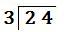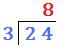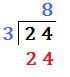### Long Division with no remainder

#### Example 1      24 ÷ 3 =      can be writen as(a)   divide 2 by 3 gives a number that is less than one so: 24 ÷ 3 = 8(b)   8 × 3 = 24(c)   24 − 24 = remainder 0So 24 ÷ 3 = 8to: